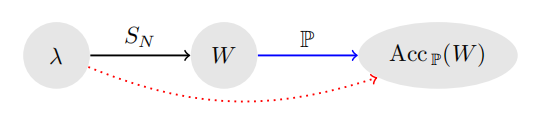# Predicting Neural Network Accuracy from Weights

In this paper Unterthiner et al. (2020) showed empirically that we can predict the generalization gap of a neural network by only looking at its weights. In this work, they released a dataset of 120k convolutional neural networks trained on different datasets.Diagram of the learning setting. Nodes contain hyperparameters λ, CNN weights W, and expected accuracy Acc P(W). Edges are labeled with the information necessary for the mapping: the training dataset SN and the data-generating distribution P

The authors extracted a set of statistics features from the network’s weights and fed it to an estimator $$\hat{F}$$ to rpedict the generalization gap(check Predict the generalization gap using marginal distribution).

### Introduction

The proposed framework relies on the encoding of the training dataset features into the network’s weights $$W_1 \cdots W_L$$. Using only the encoded features into the weights, we can extract information about how certain the network is on its encoded feature. Hence, we can use it as a feature vector fed to the estimator $$\hat{F}$$. The authors tried to investigate whether the hyper-parameters could be used only as a feature vector to our estimator.

They found that there exists a mapping from the hyper-parameters used during the training to the generalization gap if we fix the random seed and the training set. Also, Unterthiner et al. (2020) tried to train the proposed estimator on a set of CNNs trained on a single dataset and tested the estimator on another set of CNNs trained on a different dataset. They called this setting the domain shift.

### Features extracted

Now let’s start by showing the set of features extracted. First problem would be to combine the features from variable depth DNNs . The authors proposed to extract some statistics from only the input and the top three hidden layers, which showed better results than statistics from flattening the whole set of weights.

The statistics $$\tilde{W}$$ per layer includes the mean, variance and qth percentile $$q \in \{0, 25, 50, 75, 100\}$$. Extracting these statistics for both kernels and biases for the first four layers would result in a feature vector of $$4 \times 2 \times 7 = 56$$ real values. Finally, the authors fed the extracted features into a gradient boosting model and showed promising results on predicting the generalization gap using domain shift setting and different architectures in the train and test set.

#### Conclusion

From this paper, the main insight would be the possibility of extracting some meaningful information about the training set like its uncertainty and generalization gap using simply the neural network’s trained weights.

#### Side Note

I would recommend reading the paper itself and checking the related work, this is just a summary to give you a rough idea of what is going on.

• T. Unterthiner, D. Keysers, S. Gelly, O. Bousquet, and I. Tolstikhin, “Predicting Neural Network Accuracy from Weights,” arXiv:2002.11448 [cs, stat], May 2020, Accessed: Sep. 27, 2020. [Online]. Available: http://arxiv.org/abs/2002.11448.